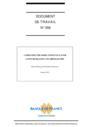# Working Paper Series no. 359: A term structure model with level factor cannot be realistic and arbitrage free

#### Abstract

A large part of the term structure literature interprets the first underlying factors as a level factor, a slope factor, and a curvature factor. In this paper we consider factor models interpretable as a level factor model, a level and a slope factor model, respectively. We prove that such models are compatible with no-arbitrage restrictions and the positivity of rates either under rather unrealistic conditions on the dynamic of the short term interest rate, or at the cost of explosive long-term interest rates. This introduces some doubt on the relevance of the level and slope interpretations of factors in term structure models.

Simon Dubecq and Christian Gourieroux
January 2012

Classification JEL : E43, E44, G12.

Keywords : Interest Rate, Term Structure, Affine Model, No Arbitrage, Level Factor, Slope Factor